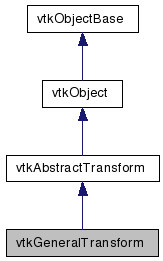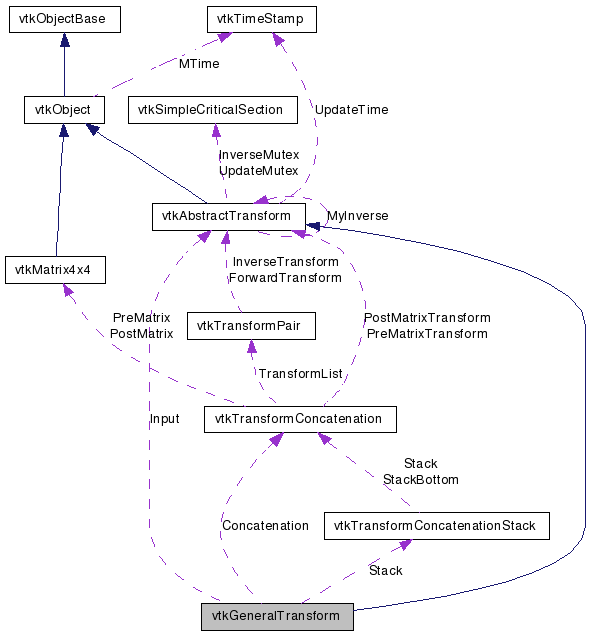vtkGeneralTransform Class Reference

#include <vtkGeneralTransform.h>

Inheritance diagram for vtkGeneralTransform:[legend]
Collaboration diagram for vtkGeneralTransform:[legend]

Detailed Description

allows operations on any transforms

vtkGeneralTransform is like vtkTransform and vtkPerspectiveTransform, but it will work with any vtkAbstractTransform as input. It is not as efficient as the other two, however, because arbitrary transformations cannot be concatenated by matrix multiplication. Transform concatenation is simulated by passing each input point through each transform in turn.

vtkTransform vtkPerspectiveTransform
Tests:
vtkGeneralTransform (Tests)

Definition at line 38 of file vtkGeneralTransform.h.

Public Types

typedef vtkAbstractTransform Superclass

Public Member Functions

virtual const char * GetClassName ()
virtual int IsA (const char *type)
void PrintSelf (ostream &os, vtkIndent indent)
void Identity ()
void Inverse ()
void Concatenate (vtkAbstractTransform *transform)
int CircuitCheck (vtkAbstractTransform *transform)
vtkAbstractTransformMakeTransform ()
unsigned long GetMTime ()
void Translate (double x, double y, double z)
void Translate (const double x)
void Translate (const float x)
void RotateWXYZ (double angle, double x, double y, double z)
void RotateWXYZ (double angle, const double axis)
void RotateWXYZ (double angle, const float axis)
void RotateX (double angle)
void RotateY (double angle)
void RotateZ (double angle)
void Scale (double x, double y, double z)
void Scale (const double s)
void Scale (const float s)
void Concatenate (vtkMatrix4x4 *matrix)
void Concatenate (const double elements)
void PreMultiply ()
void PostMultiply ()
int GetNumberOfConcatenatedTransforms ()
vtkAbstractTransformGetConcatenatedTransform (int i)
void SetInput (vtkAbstractTransform *input)
vtkAbstractTransformGetInput ()
int GetInverseFlag ()
void Push ()
void Pop ()
void InternalTransformPoint (const float in, float out)
void InternalTransformPoint (const double in, double out)
void InternalTransformDerivative (const float in, float out, float derivative)
void InternalTransformDerivative (const double in, double out, double derivative)

Static Public Member Functions

static vtkGeneralTransformNew ()
static int IsTypeOf (const char *type)
static vtkGeneralTransformSafeDownCast (vtkObject *o)

Protected Member Functions

vtkGeneralTransform ()
~vtkGeneralTransform ()
void InternalDeepCopy (vtkAbstractTransform *t)
void InternalUpdate ()

Protected Attributes

vtkAbstractTransformInput
vtkTransformConcatenationConcatenation
vtkTransformConcatenationStackStack

Member Typedef Documentation

Reimplemented from vtkAbstractTransform.

Definition at line 43 of file vtkGeneralTransform.h.

Constructor & Destructor Documentation

 vtkGeneralTransform::vtkGeneralTransform ( ) [protected]

 vtkGeneralTransform::~vtkGeneralTransform ( ) [protected]

Member Function Documentation

 static vtkGeneralTransform* vtkGeneralTransform::New ( ) [static]

Create an object with Debug turned off, modified time initialized to zero, and reference counting on.

Reimplemented from vtkObject.

 virtual const char* vtkGeneralTransform::GetClassName ( ) [virtual]

Reimplemented from vtkAbstractTransform.

 static int vtkGeneralTransform::IsTypeOf ( const char * name ) [static]

Return 1 if this class type is the same type of (or a subclass of) the named class. Returns 0 otherwise. This method works in combination with vtkTypeRevisionMacro found in vtkSetGet.h.

Reimplemented from vtkAbstractTransform.

 virtual int vtkGeneralTransform::IsA ( const char * name ) [virtual]

Return 1 if this class is the same type of (or a subclass of) the named class. Returns 0 otherwise. This method works in combination with vtkTypeRevisionMacro found in vtkSetGet.h.

Reimplemented from vtkAbstractTransform.

 static vtkGeneralTransform* vtkGeneralTransform::SafeDownCast ( vtkObject * o ) [static]

Reimplemented from vtkAbstractTransform.

 void vtkGeneralTransform::PrintSelf ( ostream & os, vtkIndent indent ) [virtual]

Methods invoked by print to print information about the object including superclasses. Typically not called by the user (use Print() instead) but used in the hierarchical print process to combine the output of several classes.

Reimplemented from vtkAbstractTransform.

 void vtkGeneralTransform::Identity ( ) [inline]

Set this transformation to the identity transformation. If the transform has an Input, then the transformation will be reset so that it is the same as the Input.

Reimplemented from vtkAbstractTransform.

Definition at line 49 of file vtkGeneralTransform.h.

 void vtkGeneralTransform::Inverse ( ) [inline, virtual]

Invert the transformation. This will also set a flag so that the transformation will use the inverse of its Input, if an Input has been set.

Implements vtkAbstractTransform.

Definition at line 54 of file vtkGeneralTransform.h.

 void vtkGeneralTransform::Translate ( double x, double y, double z ) [inline]

Create a translation matrix and concatenate it with the current transformation according to PreMultiply or PostMultiply semantics.

Definition at line 59 of file vtkGeneralTransform.h.

 void vtkGeneralTransform::Translate ( const double x ) [inline]

Create a translation matrix and concatenate it with the current transformation according to PreMultiply or PostMultiply semantics.

Definition at line 61 of file vtkGeneralTransform.h.

 void vtkGeneralTransform::Translate ( const float x ) [inline]

Create a translation matrix and concatenate it with the current transformation according to PreMultiply or PostMultiply semantics.

Definition at line 62 of file vtkGeneralTransform.h.

 void vtkGeneralTransform::RotateWXYZ ( double angle, double x, double y, double z ) [inline]

Create a rotation matrix and concatenate it with the current transformation according to PreMultiply or PostMultiply semantics. The angle is in degrees, and (x,y,z) specifies the axis that the rotation will be performed around.

Definition at line 70 of file vtkGeneralTransform.h.

 void vtkGeneralTransform::RotateWXYZ ( double angle, const double axis ) [inline]

Create a rotation matrix and concatenate it with the current transformation according to PreMultiply or PostMultiply semantics. The angle is in degrees, and (x,y,z) specifies the axis that the rotation will be performed around.

Definition at line 72 of file vtkGeneralTransform.h.

 void vtkGeneralTransform::RotateWXYZ ( double angle, const float axis ) [inline]

Create a rotation matrix and concatenate it with the current transformation according to PreMultiply or PostMultiply semantics. The angle is in degrees, and (x,y,z) specifies the axis that the rotation will be performed around.

Definition at line 74 of file vtkGeneralTransform.h.

 void vtkGeneralTransform::RotateX ( double angle ) [inline]

Create a rotation matrix about the X, Y, or Z axis and concatenate it with the current transformation according to PreMultiply or PostMultiply semantics. The angle is expressed in degrees.

Definition at line 82 of file vtkGeneralTransform.h.

 void vtkGeneralTransform::RotateY ( double angle ) [inline]

Create a rotation matrix about the X, Y, or Z axis and concatenate it with the current transformation according to PreMultiply or PostMultiply semantics. The angle is expressed in degrees.

Definition at line 83 of file vtkGeneralTransform.h.

 void vtkGeneralTransform::RotateZ ( double angle ) [inline]

Create a rotation matrix about the X, Y, or Z axis and concatenate it with the current transformation according to PreMultiply or PostMultiply semantics. The angle is expressed in degrees.

Definition at line 84 of file vtkGeneralTransform.h.

 void vtkGeneralTransform::Scale ( double x, double y, double z ) [inline]

Create a scale matrix (i.e. set the diagonal elements to x, y, z) and concatenate it with the current transformation according to PreMultiply or PostMultiply semantics.

Definition at line 91 of file vtkGeneralTransform.h.

 void vtkGeneralTransform::Scale ( const double s ) [inline]

Create a scale matrix (i.e. set the diagonal elements to x, y, z) and concatenate it with the current transformation according to PreMultiply or PostMultiply semantics.

Definition at line 93 of file vtkGeneralTransform.h.

 void vtkGeneralTransform::Scale ( const float s ) [inline]

Create a scale matrix (i.e. set the diagonal elements to x, y, z) and concatenate it with the current transformation according to PreMultiply or PostMultiply semantics.

Definition at line 94 of file vtkGeneralTransform.h.

 void vtkGeneralTransform::Concatenate ( vtkMatrix4x4 * matrix ) [inline]

Concatenates the matrix with the current transformation according to PreMultiply or PostMultiply semantics.

Definition at line 100 of file vtkGeneralTransform.h.

 void vtkGeneralTransform::Concatenate ( const double elements ) [inline]

Concatenates the matrix with the current transformation according to PreMultiply or PostMultiply semantics.

Definition at line 102 of file vtkGeneralTransform.h.

 void vtkGeneralTransform::Concatenate ( vtkAbstractTransform * transform )

Concatenate the specified transform with the current transformation according to PreMultiply or PostMultiply semantics. The concatenation is pipelined, meaning that if any of the transformations are changed, even after Concatenate() is called, those changes will be reflected when you call TransformPoint().

 void vtkGeneralTransform::PreMultiply ( ) [inline]

Sets the internal state of the transform to PreMultiply. All subsequent operations will occur before those already represented in the current transformation. In homogeneous matrix notation, M = M*A where M is the current transformation matrix and A is the applied matrix. The default is PreMultiply.

Definition at line 119 of file vtkGeneralTransform.h.

 void vtkGeneralTransform::PostMultiply ( ) [inline]

Sets the internal state of the transform to PostMultiply. All subsequent operations will occur after those already represented in the current transformation. In homogeneous matrix notation, M = A*M where M is the current transformation matrix and A is the applied matrix. The default is PreMultiply.

Definition at line 130 of file vtkGeneralTransform.h.

 int vtkGeneralTransform::GetNumberOfConcatenatedTransforms ( ) [inline]

Get the total number of transformations that are linked into this one via Concatenate() operations or via SetInput().

Definition at line 138 of file vtkGeneralTransform.h.

 vtkAbstractTransform* vtkGeneralTransform::GetConcatenatedTransform ( int i ) [inline]

Get one of the concatenated transformations as a vtkAbstractTransform. These transformations are applied, in series, every time the transformation of a coordinate occurs. This method is provided to make it possible to decompose a transformation into its constituents, for example to save a transformation to a file.

Definition at line 149 of file vtkGeneralTransform.h.

 void vtkGeneralTransform::SetInput ( vtkAbstractTransform * input )

Set the input for this transformation. This will be used as the base transformation if it is set. This method allows you to build a transform pipeline: if the input is modified, then this transformation will automatically update accordingly. Note that the InverseFlag, controlled via Inverse(), determines whether this transformation will use the Input or the inverse of the Input.

 vtkAbstractTransform* vtkGeneralTransform::GetInput ( ) [inline]

Set the input for this transformation. This will be used as the base transformation if it is set. This method allows you to build a transform pipeline: if the input is modified, then this transformation will automatically update accordingly. Note that the InverseFlag, controlled via Inverse(), determines whether this transformation will use the Input or the inverse of the Input.

Definition at line 170 of file vtkGeneralTransform.h.

 int vtkGeneralTransform::GetInverseFlag ( ) [inline]

Get the inverse flag of the transformation. This controls whether it is the Input or the inverse of the Input that is used as the base transformation. The InverseFlag is flipped every time Inverse() is called. The InverseFlag is off when a transform is first created.

Definition at line 178 of file vtkGeneralTransform.h.

 void vtkGeneralTransform::Push ( ) [inline]

Pushes the current transformation onto the transformation stack.

Definition at line 184 of file vtkGeneralTransform.h.

 void vtkGeneralTransform::Pop ( ) [inline]

Deletes the transformation on the top of the stack and sets the top to the next transformation on the stack.

Definition at line 193 of file vtkGeneralTransform.h.

 void vtkGeneralTransform::InternalTransformPoint ( const float in, float out ) [virtual]

This will calculate the transformation without calling Update. Meant for use only within other VTK classes.

Implements vtkAbstractTransform.

 void vtkGeneralTransform::InternalTransformPoint ( const double in, double out ) [virtual]

This will calculate the transformation without calling Update. Meant for use only within other VTK classes.

Implements vtkAbstractTransform.

 void vtkGeneralTransform::InternalTransformDerivative ( const float in, float out, float derivative ) [virtual]

This will calculate the transformation as well as its derivative without calling Update. Meant for use only within other VTK classes.

Implements vtkAbstractTransform.

 void vtkGeneralTransform::InternalTransformDerivative ( const double in, double out, double derivative ) [virtual]

This will calculate the transformation as well as its derivative without calling Update. Meant for use only within other VTK classes.

Implements vtkAbstractTransform.

 int vtkGeneralTransform::CircuitCheck ( vtkAbstractTransform * transform ) [virtual]

Check for self-reference. Will return true if concatenating with the specified transform, setting it to be our inverse, or setting it to be our input will create a circular reference. CircuitCheck is automatically called by SetInput(), SetInverse(), and Concatenate(vtkXTransform *). Avoid using this function, it is experimental.

Reimplemented from vtkAbstractTransform.

 vtkAbstractTransform* vtkGeneralTransform::MakeTransform ( ) [virtual]

Make another transform of the same type.

Implements vtkAbstractTransform.

 unsigned long vtkGeneralTransform::GetMTime ( ) [virtual]

Override GetMTime to account for input and concatenation.

Reimplemented from vtkAbstractTransform.

 void vtkGeneralTransform::InternalDeepCopy ( vtkAbstractTransform * ) [protected, virtual]

Perform any subclass-specific DeepCopy.

Reimplemented from vtkAbstractTransform.

 void vtkGeneralTransform::InternalUpdate ( ) [protected, virtual]

Perform any subclass-specific Update.

Reimplemented from vtkAbstractTransform.

Member Data Documentation

 vtkAbstractTransform* vtkGeneralTransform::Input [protected]

Definition at line 235 of file vtkGeneralTransform.h.

Definition at line 236 of file vtkGeneralTransform.h.

 vtkTransformConcatenationStack* vtkGeneralTransform::Stack [protected]

Definition at line 237 of file vtkGeneralTransform.h.

The documentation for this class was generated from the following file:

Generated on Wed Jun 3 19:03:50 2009 for VTK by1.5.6